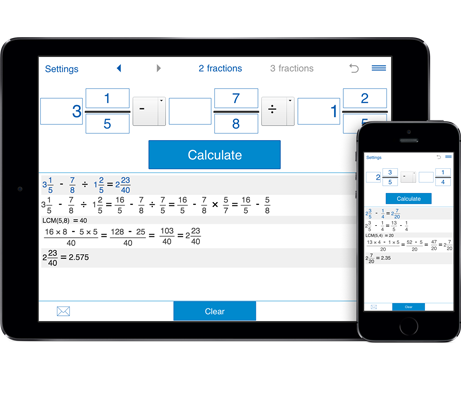# Fraction Calculator 4-in-1

Comprehensive fraction app - ideal for engineers, carpenters, builders, and everyone who needs to calculate, convert, compare and simplify fractions and mixed numbers on a daily basis.
Thanks to various options and intuitive design the calculator will be a great help for students to learn fraction arithmetic with step-by-step instructions, check math homework and train math skills.

## Quick help for fraction calculation and conversion needs

- Clear design and extensive functionality.
- Calculate, convert, compare and simplify fractions on the fly.
- Get guided explanations for your fraction problems.
- Do math with proper and improper fractions and mixed numbers, including positive and negative values.
- Math on fractions with like and unlike denominators.
- History list to review the previous entries.
- Get answers in a fractional and decimal form.
- Share calculations via email.

### Learn how to use to use the fraction converter and calculator app

#### How to add, subtract, multiply and divide fractions

You can use Fraction Calculator 4in1 to perform arithmetic operations on two and three fractions.

1. Choose "Fraction Calculator".

2. In the top menu, select the number of fractions you want to calculate. To calculate 3 fractions, you need to rotate your device into landscape mode.

3. Input the fractions. To enter negative values, just click the +/- button on the app’s keyboard.

4. Choose the operation(s) you want to perform from the drop-down menu - addition, subtraction, multiplication or division.

5. Click = and get the result in both decimal and fractional forms. Fractional results could be rounded to the nearest 1/2, 1/4, 1/8, 1/16, 1/32, 1/64, 1/128, 1/256. Decimal results can be rounded to 0-8 decimal places. If you need to get results in whole numbers, just press 0 - for decimal places and ‘-‘ for fractions.

The app shows you the step-by-step explanation of your problem, including finding the LCM for denominators and reducing the result to lowest terms.

#### More Fraction Calculators

For those who need to do math with both decimals and fractions and solve expressions with parentheses, exponents, square root, and trigonometric functions, we can recommend Fraction Pro that provides the standard calculator input. The app supports nested parentheses and allows you to enter expressions of any complexity.

Need to convert between inches and feet, US standard and metric units, decimals and fractions? Try Units Master, an advanced unit converter and calculator that not only offers all the options available in Fractions Pro but also supports unit calculation and conversion.

#### How to convert fractions to decimals and vice versa

Unlike many fraction converters, Fraction Calculator 4-in-1 supports proper and improper fractions, mixed numbers, terminating and repeating decimals. In seconds, you can convert fractions into their decimal equivalents as well as decimal numbers to fractions in a reduced form. The app learns how to convert between decimals and fractions.
When converting fractions and decimals, you may want the result to be rounded. For example you may want to round results to 100ths, 8ths, 16ths, 32nds, etc. In app’s Settings, you can select the calculation precision for both fractions and decimals.

1. Choose Fractions <-> Decimals.
2. If you want to convert fractions to decimals, choose Fractions and enter the value you are going to convert.
To convert a decimal number into a fraction, press Decimals and type a decimal number using the decimal point "." as separator eg. 5.31
To convert repeating decimals enclose the repeating digits in parenthesis. Eg. if you want to convert 5.24333, type 5.24(3)

#### How to compare two or three fractions or mixed numbers

1. Choose "Compare fractions" in the main window.
2. Select 2 fractions or 3 fractions. For a mobile version, to compare three fractions you need to rotate your device into landscape mode.
3. Input fractions and click "Compare".
The application will show you the result and detailed explanation, including finding the LCM (Lowest Common Denominator).

#### How to simplify fractions

The calculator simplifies fractions and mixed numbers and shows the work involved.
Select "Simplifying fractions", enter a fraction into the input field and press =. You will see the result in the output window.

#### Fraction Calculator 4in1 FAQ

- What fraction problems can I solve using Fraction Converter Calculator?
The app provides detailed solutions for fraction addition, subtraction, multiplication, division, conversion, comparison, and simplification.

- How do I print my calculations?
At this moment, there is no special option to print your calculations. But you can easily re-send your calculations via email and then print them out.

- How do I view, access and edit my previous solved problems?
Use the left arrow and right arrow buttons in the top menu to move between recent calculations. As soon as you found the problem, press the equal button to show the details. To edit the problem, just input new values into the fields and/or change the operations.
The history button (anticlockwise open circle arrow) opens the list of solved problems shorten to an expression and its result.